# Descriptive Statistics

## Objective

Describe the shape of the data in box plots and histograms. Choose an appropriate measure of center (or an appropriate shape) based on the shape and the relationship between the mean and the median.

## Common Core Standards

### Core Standards

?

• HSS-ID.A.2 — Use statistics appropriate to the shape of the data distribution to compare center (median, mean) and spread (interquartile range, standard deviation) of two or more different data sets.

• HSS-ID.A.3 — Interpret differences in shape, center, and spread in the context of the data sets, accounting for possible effects of extreme data points (outliers).

?

• 6.SP.B.5

• 7.SP.B.3

## Criteria for Success

?

1. Understand that shape describes the skew of a data set. Describe a data set as skew right, symmetrical, or skew left, depending on the relationship of the mean to the median of a data set.
2. Describe how outliers affect the mean and the median, and how this appears in the shape of the graph.
3. Describe that a symmetrical shape is when the mean and the median are equal and is loosely referred to as a "normal distribution."
4. Identify median as “outlier resistant,” which means that it does not change when a data item is replaced by an outlier.
5. Describe the shape of a data set where the mean is greater than the median as skew right, or positively skewed.
6. Describe the shape of a data set where the median is greater than the mean as skew left, or negatively skewed.

## Tips for Teachers

?

• This is a great visual of how mean and median relate in different skew situations.
• What Is a p-Value Anyway? Andrew J. Vickers, Pearson (2009). Chapter 2 is especially helpful.
• This GeoGebra tool is used in later lessons to discuss the standard deviation but is very helpful in visualizing what it means for median to be “outlier resistant.”

## Anchor Problems

?

### Problem 1

How are these three graphs different?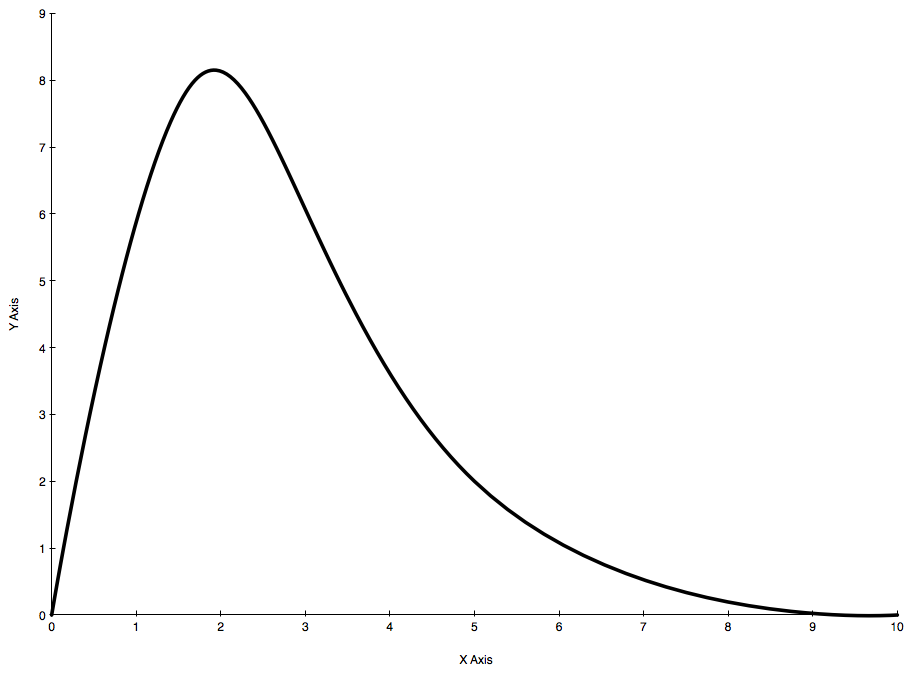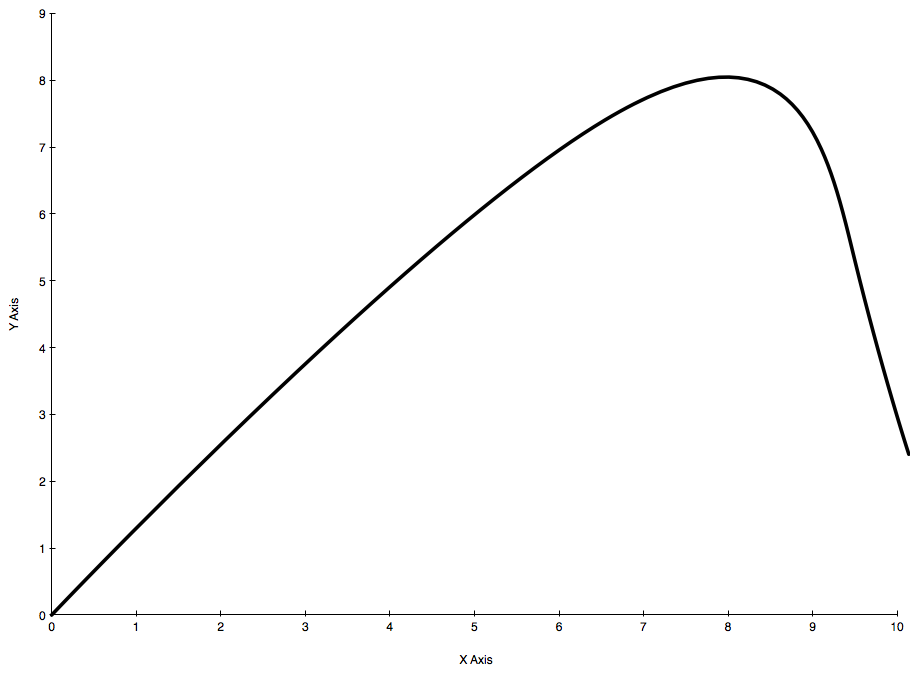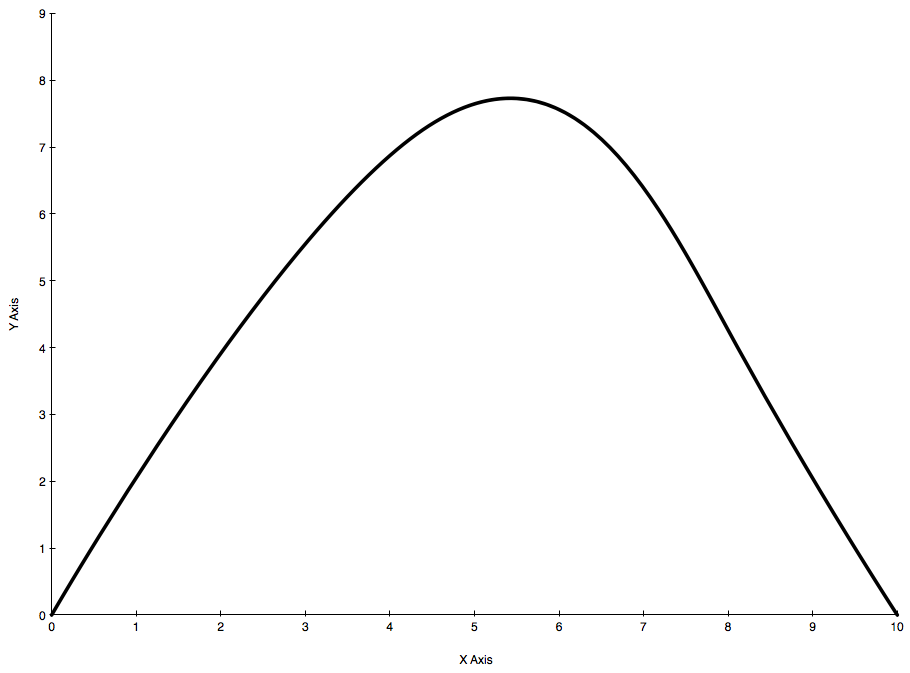### Problem 2

Below is a histogram representing the total assessed property value of single-family houses in Boston during fiscal year 2017.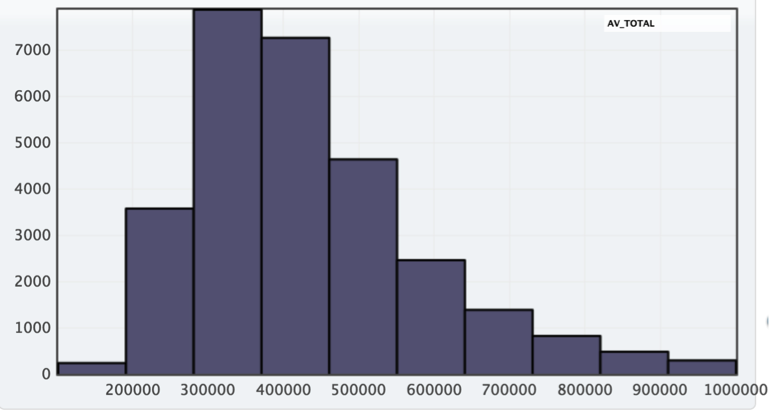The graph does not include houses greater than $1,000,000 in assessed value. The descriptive data provided does include the houses greater than$1,000,000 in assessed value.

Approximate where you think the mean and the median would be based on the shape of the data.

#### References

Analyze Boston Property AssessmentFY2017 property data from the city of Boston

Property Assessment published by Department of Innovation and Technology is made available by Analyze Boston under the Open Data Commons PDDL. Accessed June 26, 2017, 2:31 p.m..

### Problem 3

Below is a box plot of data that describes the amount of money spent per student on average, by state, in the school year 2004–2005.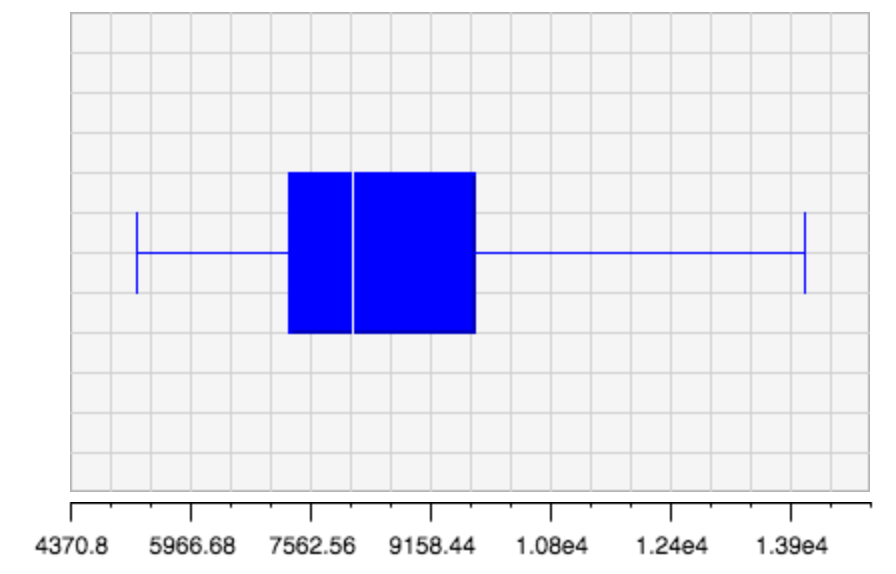Explain, is this data skew left, right, or symmetrical?

#### References

Shodor Interactivate Box Plots, Amount Spent Per Student

Box Plots, Amount Spent Per Student is made available by Shodor Education Foundation, Inc. on Shodor Interactivate. ©1994-2018 The Shodor Education Foundation, Inc. Accessed June 26, 2017, 2:37 p.m..

## Problem Set

?

The following resources include problems and activities aligned to the objective of the lesson that can be used to create your own problem set.

• Use mean and median to determine the skew of the data set (without the graph). Justify reasoning with a dot plot of the data. This should come after a couple of lower-rigor problems in the beginning of the problem set.
• Error analysis with right skew going the wrong direction
• Review of previous lessons of center
• Ask students to describe the shape and center of the data so that tomorrow they can dive into the spread.

?

Each person in a random sample of ten ninth graders was asked one question:

• How many hours did you spend watching TV last night?

Here are the data for these ten students: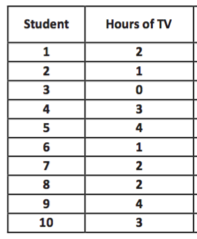1. Construct a box plot and a dot plot for the data for these ten students. Would you describe this data distribution as symmetric or as skewed?
2. If you wanted to describe a typical number of hours of TV for these ten students, would you use the mean or the median? Calculate the value of the measure you selected.